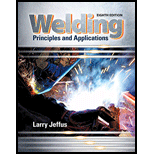Chapter 20, Problem 17R### Welding: Principles and Applicatio...

8th Edition
Larry Jeffus
ISBN: 9781305494695

#### Solutions

Chapter
Section### Welding: Principles and Applicatio...

8th Edition
Larry Jeffus
ISBN: 9781305494695
Textbook Problem
1 views

# How many total feet of metal stock would you need if one piece is 12 ft 5 in. long and the other is 7 ft 3 in. long?

To determine

Length of the total metal required.

Explanation

Given information:

Length of the one piece, l1=12 ft 5 in.

Length of the other piece, l2=7 ft 3 in.

Calculation:

Total length of the metal piece is the summation of length of the two pieces,

L=l1+l2L=12ft5in.+7ft3in.L=(12+7)ft(5+3)in

### Still sussing out bartleby?

Check out a sample textbook solution.

See a sample solution

#### The Solution to Your Study Problems

Bartleby provides explanations to thousands of textbook problems written by our experts, many with advanced degrees!

Get Started

#### Calculate the reaction at D for the structure described in Prob. 5.11.

International Edition---engineering Mechanics: Statics, 4th Edition

#### Briefly describe a micro drill press and its purpose.

Precision Machining Technology (MindTap Course List)

#### What is informatics?

Principles of Information Systems (MindTap Course List)

#### Describe how a decision support system (DSS) is used. OLAP stands for _____.

Enhanced Discovering Computers 2017 (Shelly Cashman Series) (MindTap Course List)

#### Compute the interest earned on the deposits made in Problem 20.1.

Engineering Fundamentals: An Introduction to Engineering (MindTap Course List)